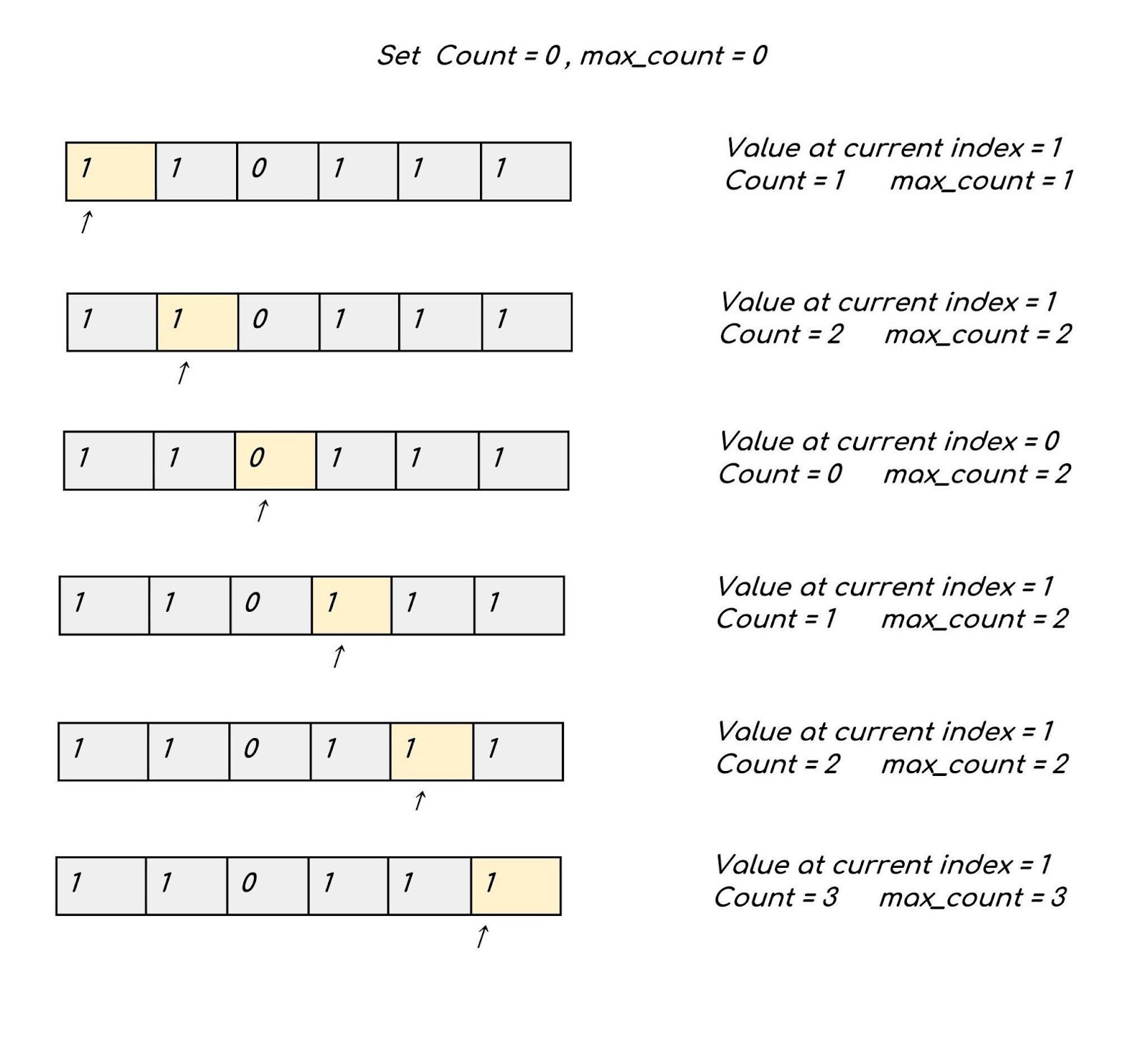# Count Maximum Consecutive One’s in the array

Problem Statement: Given an array that contains only 1 and 0 return the count of maximum consecutive ones in the array.

Examples:

```Example 1:

Input: prices = {1, 1, 0, 1, 1, 1}

Output: 3

Explanation: There are two consecutive 1’s and three consecutive 1’s in the array out of which maximum is 3.

Input: prices = {1, 0, 1, 1, 0, 1}

Output: 2

Explanation: There are two consecutive 1's in the array.
```

Solution:

Disclaimer: Don’t jump directly to the solution, try it out yourself first.

Approach:  We maintain a variable count that keeps a track of the number of consecutive 1’s while traversing the array. The other variable max_count maintains the maximum number of 1’s, in other words, it maintains the answer.

We start traversing from the beginning of the array. Since we can encounter either a 1 or 0 there can be two situations:-

1. If  the value at the current index is equal to 1 we increase the value of count by one. After updating  the count variable if it becomes more than the max_count  update the max_count.
2. If the value at the current index is equal to zero we make the variable count as 0 since there are no more consecutive ones.

See the illustration below for a better understandingCode:

## C++ Code

``````#include <bits/stdc++.h>

using namespace std;
class Solution {
public:
int findMaxConsecutiveOnes(vector < int > & nums) {
int cnt = 0;
int maxi = 0;
for (int i = 0; i < nums.size(); i++) {
if (nums[i] == 1) {
cnt++;
} else {
cnt = 0;
}

maxi = max(maxi, cnt);
}
return maxi;
}
};

int main() {
vector < int > nums = { 1, 1, 0, 1, 1, 1 };
Solution obj;
int ans = obj.findMaxConsecutiveOnes(nums);
cout << "The maximum  consecutive 1's are " << ans;
return 0;
}``````

Output: The maximum consecutive 1’s are 3.

Time Complexity: O(N) since the solution involves only a single pass.

Space Complexity: O(1) because no extra space is used.

## Java Code

``````import java.util.*;
public class Main {
static int findMaxConsecutiveOnes(int nums[]) {
int cnt = 0;
int maxi = 0;
for (int i = 0; i < nums.length; i++) {
if (nums[i] == 1) {
cnt++;
} else {
cnt = 0;
}

maxi = Math.max(maxi, cnt);
}
return maxi;
}
public static void main(String args[]) {
int nums[] = { 1, 1, 0, 1, 1, 1 };
int ans = findMaxConsecutiveOnes(nums);
System.out.println("The maximum  consecutive 1's are " + ans);
}
}``````

Output: The maximum consecutive 1’s are 3.

Time Complexity: O(N) since the solution involves only a single pass.

Space Complexity: O(1) because no extra space is used.